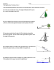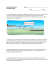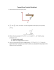# trigonometry applications in right triangles - pdf

## Transcription

trigonometry applications in right triangles - pdf
```Geometry – Unit 6 Practice
Trigonometry Applications in Right Triangles
G.SRT.C.8
Name: _____________________________!
Date: ___________ Pd: ____
1) The figure shown is a trapezoid. Using the information given, find the area of
this trapezoid to the nearest square unit. The area formula for a trapezoid is
A=
( b1 + b2 ) h .
2
2) A 16 foot ladder is leaning against a house. It touches the bottom of a window that is 12 feet 6 inches
above the ground. What is the measure of the angle that the ladder forms with the ground? Round to
the nearest 10th of a degree.
3) Haley is flying a kite whose string is making a 70° angle with the ground. The kite string is 65 meters
long. How far is the kite above the ground?
4) A surveyor is 100 meters from the base of a dam. The angle of elevation to the top of the dam measures
26° . The surveyor's eye-level is 1.73 meters above the ground. Find the height of the dam to the
nearest hundredth of a meter.
5) Two boats leave the same port. One goes 10 miles due west and drops
anchor. The other leaves the port 20 degrees north of west. How far
must it go in a straight line to get as far west as the first boat?
6) A girl flies a kite with a 100 foot string. The angle of elevation of the
string is 52o. How high off the ground is the kite?
7) An airplane takes off 200 yards in front of a 60 foot
building. At what angle of elevation must the plane take
off in order to avoid crashing into the building? Assume
that the airplane flies in a straight line and the angle of
elevation remains constant until the airplane flies over
the building.
8) A 16 foot ladder is used to scale a 13 foot wall. At what angle of elevation
must the ladder be situated in order to reach the top of the wall?
According to ladder safety sources, the distance from the ladder's base to
the wall should equal one-fourth the distance from the base of the wall to
the point where the ladder touches the wall. Find y and determine if the
placement of this ladder is safe.
SNRPDP
Unit 6: Right Triangles & Trigonometry
NVACS – Revised 2014-2015
16
y
Page 1 of 4
Practice – Unit 6 (cont.)
9) A ramp is needed to allow vehicles to climb a 2 foot wall. The angle of
elevation in order for the vehicles to safely go up must be 30o or less, and
the longest ramp available is 5 feet long. Can this ramp be used safely?
10) Grain elevators are conveyor belts used throughout the Midwest to take produce,
like wheat, to the top of a silo for storage. How long would the belt of a grain
elevator need to be if the bottom is 50 feet from the base of a silo that is 50 feet
11) If the angle of elevation to the top of a tree is 20°. How far are you away from the tree, if the tree's
12) Which statement can NOT be used to find the length of x?
6
A) tan16 =
x
x
B) cos16 =
21
x
C) tan 74 =
6
x
D) sin16 =
6
13) In the figure, ABCD is a rectangle whose perimeter is 30. The length of BE is 12.
Find to the nearest degree, the measure of angle E.
14) Anne sees a rocket at an angle of elevation of 22 degrees. If the rocket launch pad is 5 miles away
from Anne, then how high is the rocket?
15) A flagpole is 18 feet high. The flagpole casts a shadow of 24 feet along the ground. Calculate the
distance between the end of the shadow and the top of the flag pole and calculate the angle between
the shadow and the line representing the distance.
16) In sailing, it is not possible to head straight into the wind. In order to get upwind, a
sailboat simply sails at an angle to the wind and then turns to sail back toward where it
wants to go. Assume that a boat needs to get directly upwind 6000 m. If it sails off at a
45° angle to the wind and then turns back 90° towards the original goal, how far would it
have to sail to get directly upwind 6000 meters? Round your answer to the nearest
tenth.
17) A UFO is hovering 800 feet above a road. A truck driver observes the UFO at a twenty degree
angle. Twenty five seconds later the truck driver notices the angle of the UFO is now at sixty
degrees. How fast is the truck moving? Round your answer to the nearest integer (feet per
second).
Page 2 of 4
Practice – Unit 6 (cont.)
18) A large airplane flying at 26,000 feet sights a smaller plane traveling at an altitude of 24,000 feet. The
angle of depression is 40°. What is the line of sight distance between the two planes?
19) A woodcutter wants to determine the height of a tall tree. He stands at some distance from the tree and
determines that the angle of elevation to the top of the tree is 40°. He moves 30′ closer to the tree, and
now the angle of elevation is 50°. If the woodcutter's eyes are 5′ above the ground, how tall is the tree?
20) A ladder must reach the top of a building. The base of the ladder will be 25′ from the base of the
building. The angle of elevation from the base of the ladder to the top of the building is 64°. Find the
height of the building and the length of the ladder.
21) From the top of a cliff, 56 meters above sea-level, two buoys can
be seen which lie in a straight line with the observer. The angles of
depression of these buoys from the observer are 28° and 41°
respectively. Calculate the distance between the two buoys.
22) From the top of a barn 25 feet tall, you see a cat on the ground. The
angle of depression of the cat is 40°. How many feet, to the nearest
foot, must the cat walk to reach the barn directly below you?
23) From a point on the ground 25 feet from the foot of a tree, the angle of elevation of the top
of the tree is 32°. Find to the nearest foot, the height of the tree.
24) Roof trusses often use right triangles to make a flimsy 2 x 4 more rigid to hold up the weight of the roof.
If a house is 40 feet wide and the roof is an isosceles triangle with base angles of 30°, how far is it from
the bottom edge of the roof to the peak? Round your answer to the nearest tenth.
25) If the angle of elevation to the top of a billboard is 65°. How far are you away from the billboard, if the
billboard’s height is 27 feet? Round your answer to the nearest tenth
26) What is the height of the tree on the right? Round your answer to the nearest foot.
Page 3 of 4
Practice – Unit 6 (cont.)
27) A hiker is hiking up a 12 degree slope. If he hikes at a constant rate of 3 mph, how much altitude does he
gain in 5 hours of hiking?
28) 75 yards away from a tall post, the angle of elevation of the top of the post is 21.4°. (a) Find the height
of the post. There is a sticker on the post which is exactly half way up. From that same observation point
(b) what is the angle of elevation of that sticker?
29) Airport meteorologists keep an eye on the weather to ensure the safety of the flights. One thing they watch is
the cloud ceiling. The cloud ceiling is the lowest altitude at which solid cloud is visible. If the cloud ceiling is too
low the planes are not allowed to take off or land.
One way a meteorologist can find the cloud ceiling at
night is to shine a searchlight that is located a fixed
distance from their office vertically into the clouds.
Then they measure the angle of elevation to the spot
of light on the cloud. The angle of elevation is the
angle formed by the line of sight to the spot and the
horizontal. Using trigonometry, the cloud ceiling can
be determined
A searchlight located 200 meters from a weather office is turned on. If the angle of elevation to the spot
of light on the clouds is 35° , how high is the cloud ceiling?
30) At 57” from the base of a building you need to look up at 55° to see the top of a
building. What is the height of the building?
Can you find the mistake in the question/diagram that makes it and the answer seem
unreasonable?
Page 4 of 4
```

### Worksheet - Multi-Step Trigonometry Problem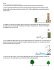### File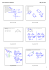### File - HHS Pre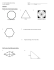### installation of an angle board * * * thermopian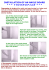### AC Geo/Adv.Alg Unit 1 Worksheet 11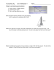### Problem Solving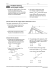### Review12.1-12.6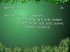### Trig Applications ws day 2 geo 14